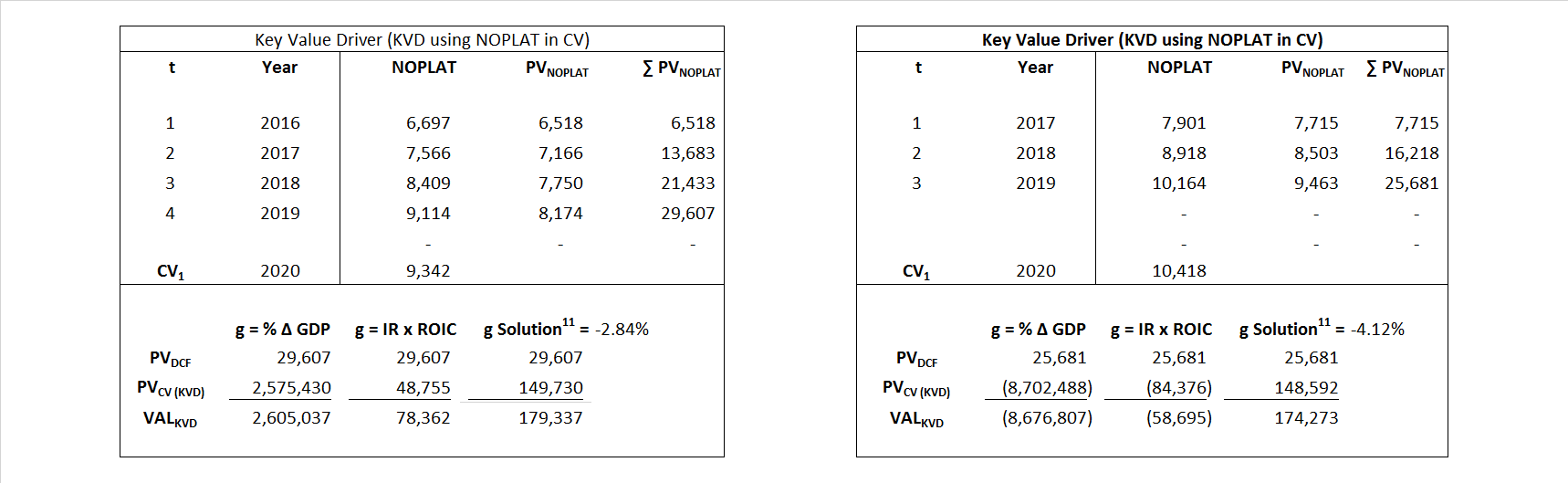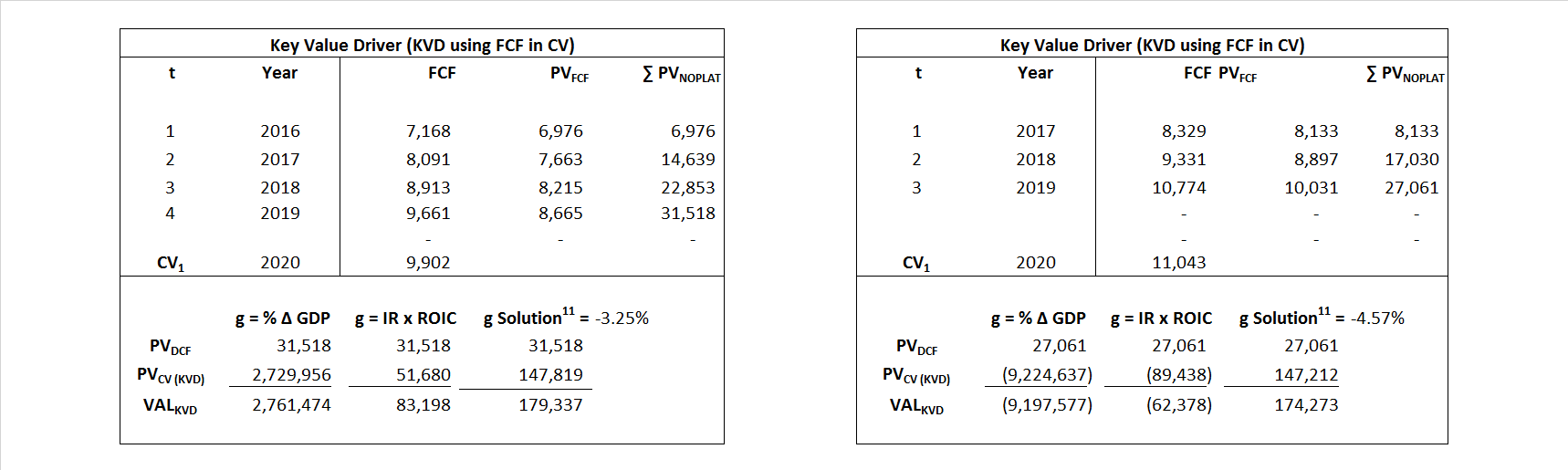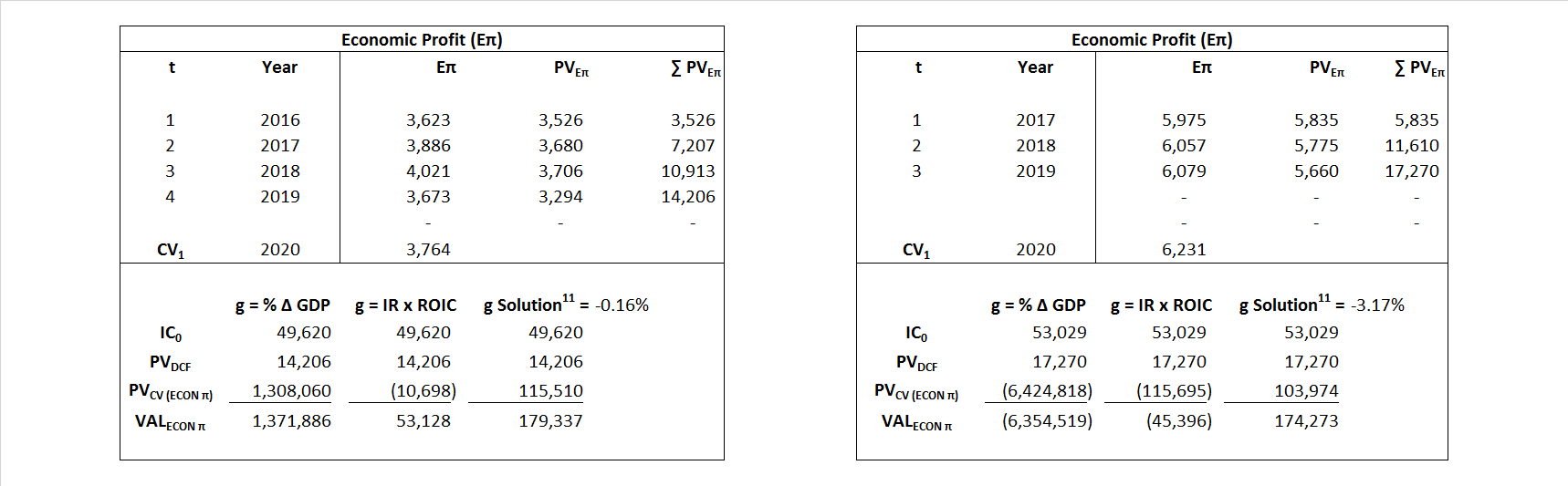# Visa

## Analyst Listing

The following analysts provide coverage for the subject firm as of May 2016:

 Broker Analyst Analyst Email SunTrust Robinson Humphrey Andrew Jeffrey andrew.jeffrey@suntrust.com Nomura Research Bill Carcache bill.carcache@nomura.com Deutsche Bank Research Bryan Keane bryan.keane@db.com Stifel Nicolaus Christopher C. Brendler ccbrendler@stifel.com Atlantic Equities Christopher Hickey c.hickey@atlantic-equities.com Sandler O’Neill & Partners Christopher R. Donat cdonat@sandleroneill.com RBC Capital Markets Daniel Perlin daniel.perlin@rbccm.com Evercore ISI David Togut david.togut@evercoreisi.com Guggenheim Securities Eric Wasserstrom eric.wasserstrom@guggenheimpartners.com Cowen & Company Georgios Mihalos george.mihalos@cowen.com Wedbush Securities Gil Luria gil.luria@wedbush.com Oppenheimer Glenn Greene glenn.greene@opco.com BMO Capital Markets James Fotheringham james.fotheringham@bmo.com Susquehanna Financial Group James Friedman james.friedman@sig.com Piper Jaffray Jason Deleeuw jason.s.deleeuw@pjc.com Jefferies Jason Kupferberg jkupferberg@jefferies.com Compass Point Research John T. Williams jwilliams@compasspointllc.com Pacific Crest Securities-KBCM Josh Beck jbeck@pacific-crest.com Bernstein Research Lisa D. Ellis lisa.ellis@bernstein.com CRT Capital Group Moshe Katri mkatri@sterneageecrt.com Credit Suisse Moshe Orenbuch moshe.orenbuch@credit-suisse.com William Blair Robert Napoli bnapoli@williamblair.com Keefe Bruyette & Woods Sanjay Sakhrani ssakhrani@kbw.com Wells Fargo Securities Timothy Willi timothy.willi@wellsfargo.com Raymond James Wayne Johnson wayne.johnson@raymondjames.com

## Primary Input Data## Derived Input Data

### Equational Form

Net Operating Profit Less Adjusted Taxes NOPLAT   6,003   6,983$NOPLAT\, =\, EBIT\, x\, (1 \,-\, Avg \,\,Tax\,\, Rate\,\, on\,\, EBIT)$
Free Cash Flow FCF  6,366  5,535$FCF\,=NOPLAT\,+\,Non-Cash\,Expenses-\Delta NWC\,-\,NCS$
Tax Shield TS  8  146$TS\,=\,Interest\,\,Paid\,\,x\,\, Avg \,\,Tax\,\,Rate\,\, on\,\, Pre-Tax\,\, Income$
Invested Capital IC  49,620  53,029$IC\,=\,Fixed\,\,Operating\,\,Assets\,\,+\,\,Net\,\, Working\,\, Capital$
Return on Invested Capital ROIC 12.10% 13.17%$ROIC\,=\,\frac { NOPLAT }{ IC }$
Net Investment NetInv   17,028  3,937$NetInv\,=\,{ {IC}_{1}}-{{IC}_{0}}+Depreciation$
Investment Rate IR 283.64% 56.38%$IR\,=\,\frac {NetInv}{NOPLAT}$
Weighted Average Cost of Capital WACCMarket 2.76% 2.41%$WACC\,=\,\frac { E }{ V } { R }_{ E }\,+\,\frac { P }{ V } { R }_{ P }\,+\,\frac { D }{ V } { R }_{ D }\left( 1- Avg\,\, Tax\,\,Rate\,\,on\,\,Pre-Tax\,\,Income \right)$
WACCBook  9.19% 8.20%
Enterprise value EVMarket  179,337  174,273$EV\,=\,Market\,\,Cap\,\,Equity\,+\,\,Long\,\,Term\,\,Debt\,-\,Cash$
EVBook  191,900  184,738
EV/EBIT Multiple$\frac{EV_{Market}}{EBIT}$  19.42  16.22$EV/EBIT\,=\,\frac { EV}{ EBIT}$
Long-Run Growth g = IR x ROIC
34.32%   7.42% Long-run growth rates of the income variable  are used in the Continuing Value portion of the valuation models.
g = %$\Delta$ GDP   2.50%   2.50%

## Valuation Model Outcomes

The outcomes presented in this study are the result of original input data, derived data, and synthesized inputs and, depending on the equational form of any particular valuation model, may result in irrelevant or implausible results.  For example, in the event WACC < g, the value of this term, often found in the denominator of an equation’s continuation value term, will be expressly negative and may result in a negative overall valuation for the firm.  In the event of a WACC < g relation, the model form as applied to the subject firm offers an irrelevant outcome.

### Equational form

Key Value Driver (NOPLAT) KVD (NOPLAT)${ Value }_{ DCF/KVD }=\sum { \frac { NOPLAT_{ t } }{ { \left( 1+WACC \right) }^{ t } } +\frac { \frac { { NOPLAT }_{ 1 }\left( 1-\frac { g }{ ROIC } \right) }{ WACC-g } }{ { \left( 1+WACC \right) }^{ t } } }$Key Value Driver (FCF) KVD (FCF)${ Value }_{ DCF/KVD }=\sum { \frac { FCF_{ t } }{ { \left( 1+WACC \right) }^{ t } } +\frac { \frac { { NOPLAT }_{ 1 }\left( 1-\frac { g }{ ROIC } \right) }{ WACC-g } }{ { \left( 1+WACC \right) }^{ t } } }$Free Cash Flow FCF${ Value }_{ DCF/FCF }=\sum { \frac { FCF_{ t } }{ { \left( 1+WACC \right) }^{ t } } +\frac { \frac { { FCF }_{ 1 }}{ WACC-g } }{ { \left( 1+WACC \right) }^{ t } } }$Economic Profit ECON π${ Value }_{ { ECON\pi } }= I{ C }_{ 0 }+\sum { \frac { { IC }_{ t-1 }(ROI{ C }_{t}-WAC{C}_{t}) }{ { \left( 1+WACC \right) }^{ t } }+ \frac {\frac { I{C}_{0}\ x\ (ROI{C}_{1}\ -\ WAC{C}_{1}) }{ WACC-g } }{ { \left( 1+WACC \right) }^{ t } } }$Adjusted Present Value APV${ Value }_{ APV }=\sum { \frac { FCF_{ t } }{ { \left( 1+{ k }_{ u } \right) }^{ t } } +\frac { \frac { { FCF }_{ 1 }}{ { k }_{ u }-g } }{ { \left( 1+{ k }_{ u } \right) }^{ t } } } +\sum { \frac { { TS }_{ t } }{ { \left( 1+{ k }_{ tax } \right) }^{ t } } +\frac { \frac { { TS }_{ 1 }}{ { k }_{ tax }-g } }{ { \left( 1+{ k }_{ tax } \right) }^{ t } } }$Forward Market Multiple FMM${ Value }_{ DCF/FMM}=\sum { \frac { FCF_{ t } }{ { \left( 1+WACC \right) }^{ t } } +\frac { { EBIT }_{ 1 }\,{x}\,{FMM}}{ { \left( 1+WACC \right) }^{ t } } }{\,\,\,; \,\,FMM\,=\,\frac{{EV}_{t=0}}{{EBIT}_{t=0}}}$